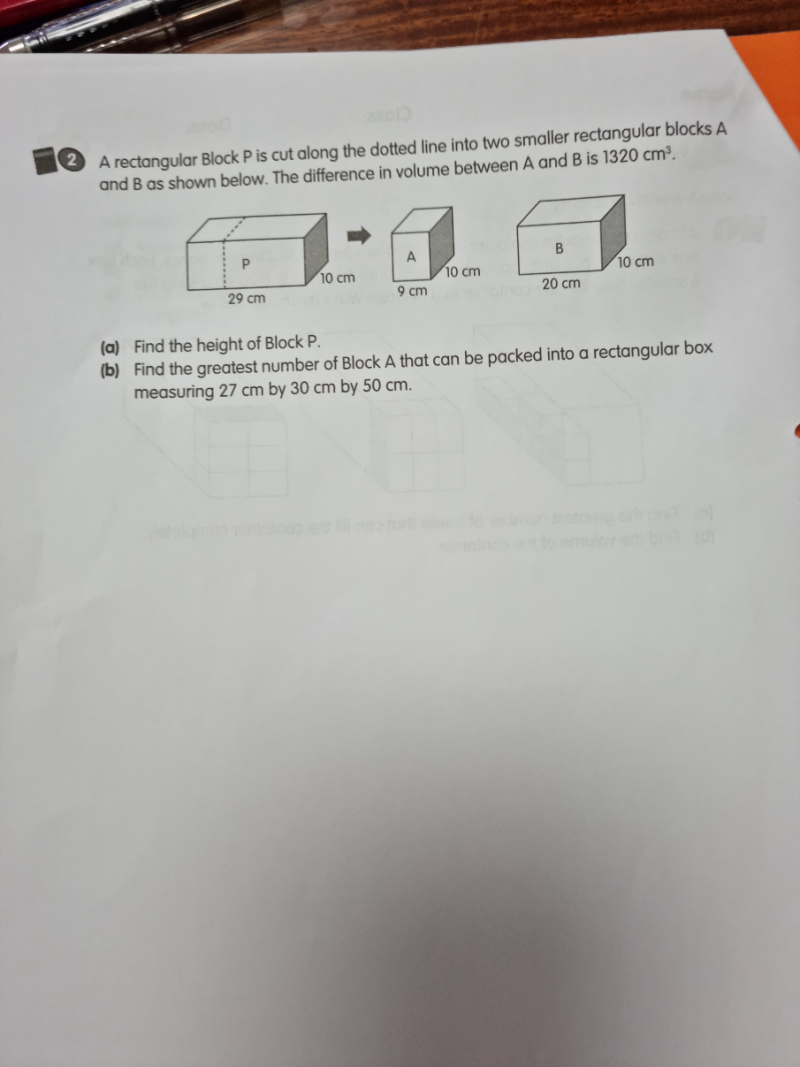# QuestionThank you.

(a) Difference in breadth in the blocks A & B = 20-9 = 11cm

h = height of block P, A and B

Difference in volume = 11 x 10 x h = 1320

Therefore h = 1320/110 = 12cm

Volume of block A = 10 x 9  x 12 = 1080

Volume of rectangular box = 27 x 30 x 50 = 40500

40500/1080 = 37.5

Therefore, maximum number of block A packed into rectangular box = 37